## RS Aggarwal Class 10 Solutions Chapter 10 Quadratic Equations Ex 10C

These Solutions are part of RS Aggarwal Solutions Class 10. Here we have given RS Aggarwal Solutions Class 10 Chapter 10 Quadratic Equations Ex 10C.

Other Exercises

Find the discriminant of each of the following equations:
Question 1.
Solution: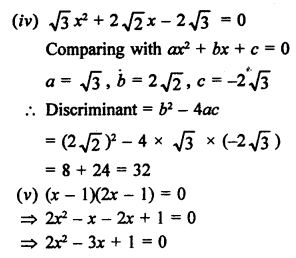Find the roots of each of the following equations, if they exist, by applying the quadratic formula:
Question 2.
Solution: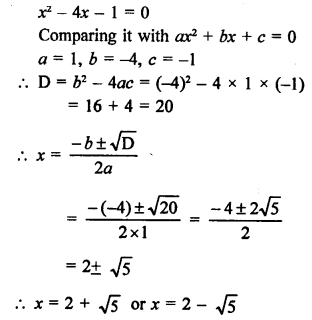Question 3.
Solution: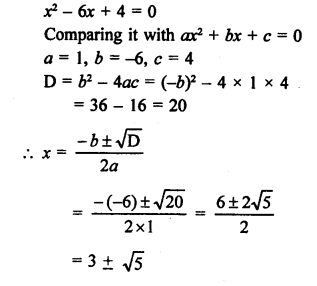x = 3 + √5 or x = 3 – √5

Question 4.
Solution: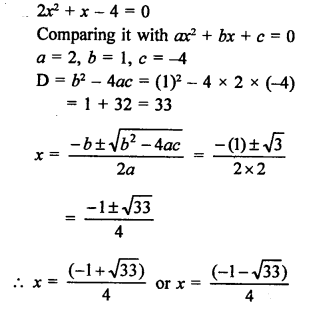Question 5.
Solution: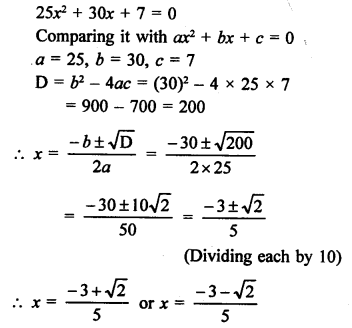Question 6.
Solution: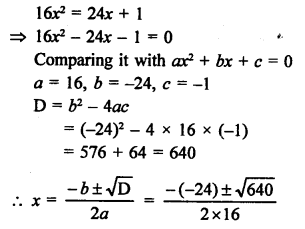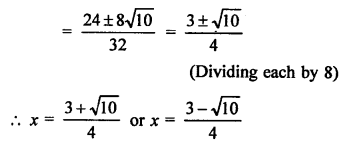Question 7.
Solution: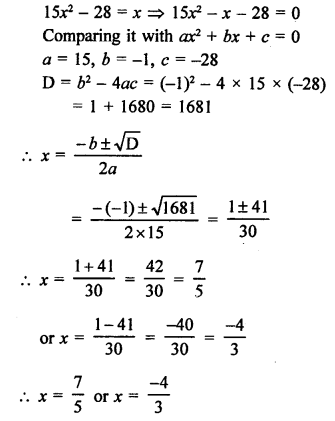Question 8.
Solution:Question 9.
Solution: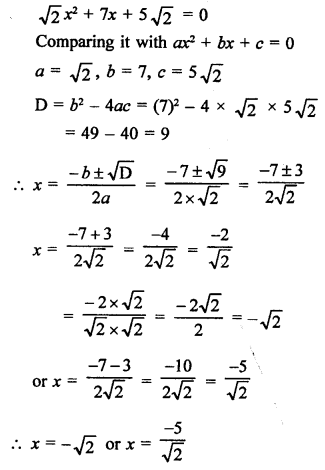Question 10.
Solution: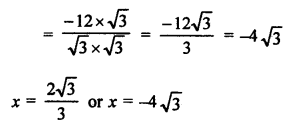Question 11.
Solution: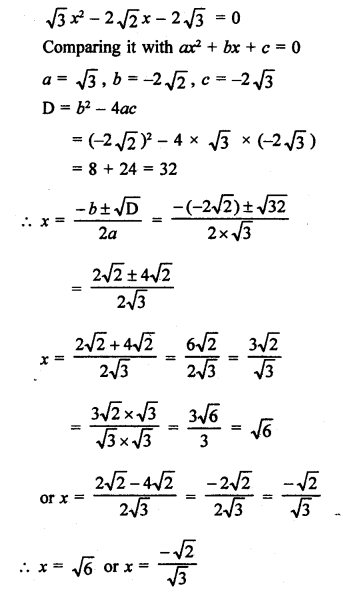Question 12.
Solution: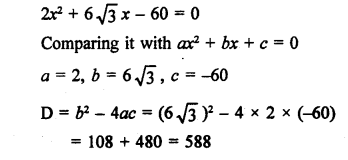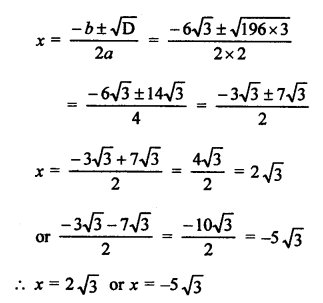Question 13.
Solution: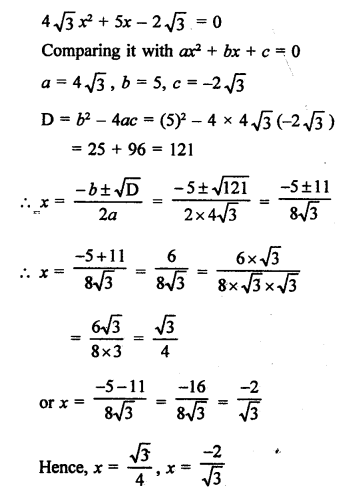Question 14.
Solution: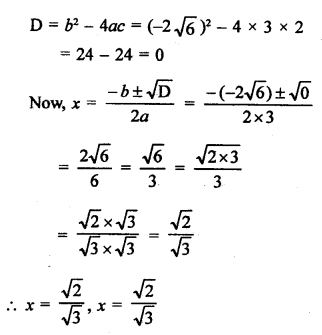Question 15.
Solution:Question 16.
Solution:
x² + x + 2 = 0
Comparing it with ax² + bx + c = 0
a = 1, b = 1, c = 2
D = b² – 4ac = (1)² – 4 x 1 x 2 = 1 – 8 = -7
D < 0
There are no real roots.

Question 17.
Solution:Question 18.
Solution: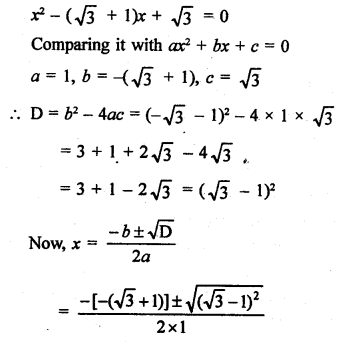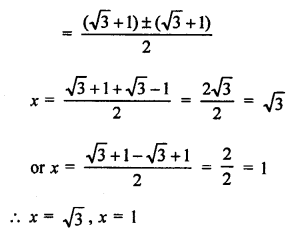Question 19.
Solution:Question 20.
Solution:
3x² – 2x + 2 = 0
Comparing it with ax² + bx + c = 0
a = 3, b = -2, c = 2
D = b² – 4ac = (-2 )² – 4 x 3 x 2 = 4 – 24 = -20
D < 0
Roots are not real.

Question 21.
Solution: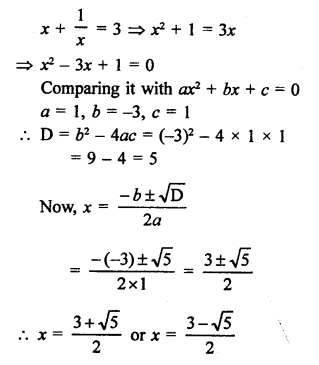Question 22.
Solution: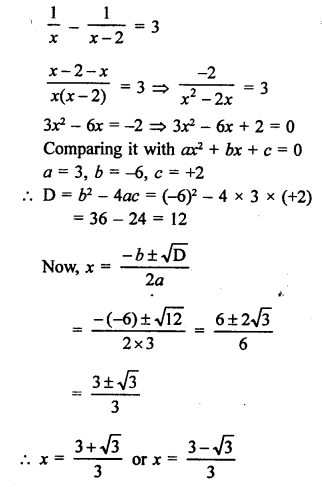Question 23.
Solution: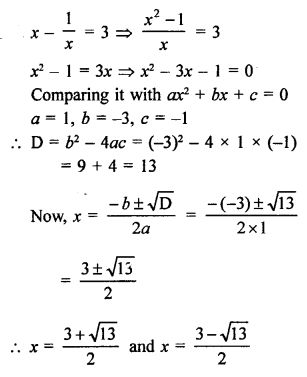Question 24.
Solution: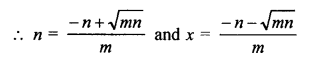Question 25.
Solution: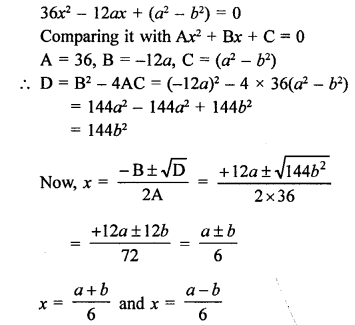Question 26.
Solution: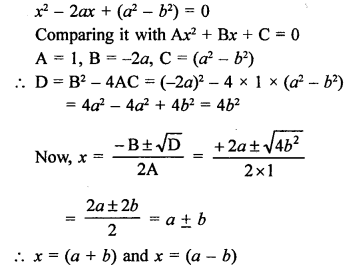Question 27.
Solution: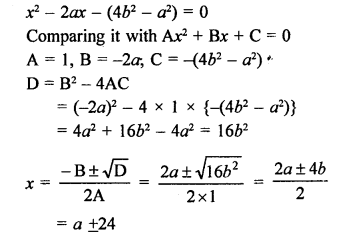x = (a + 2b) and x = (a – 2b)

Question 28.
Solution: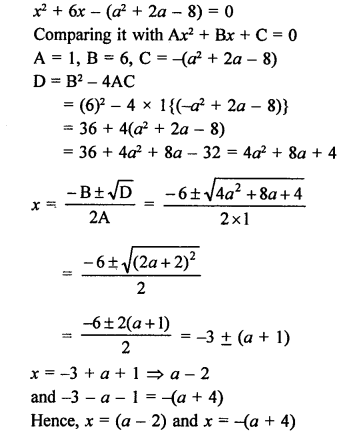Question 29.
Solution: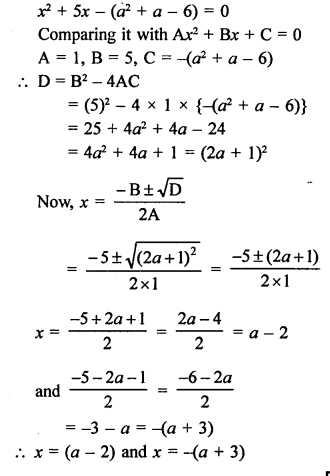Question 30.
Solution: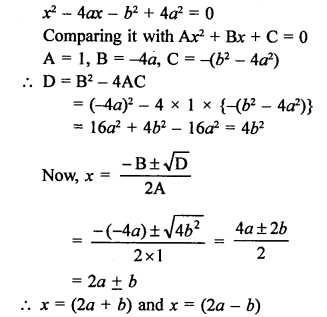Question 31.
Solution:Question 32.
Solution: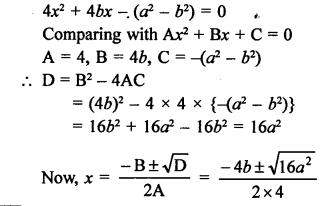Question 33.
Solution:Question 34.
Solution: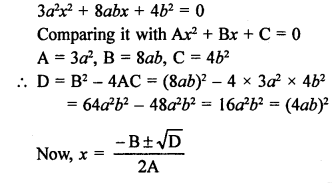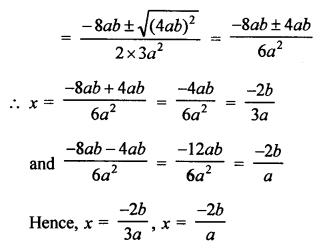Question 35.
Solution: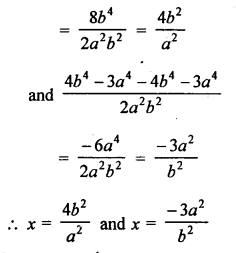Question 36.
Solution: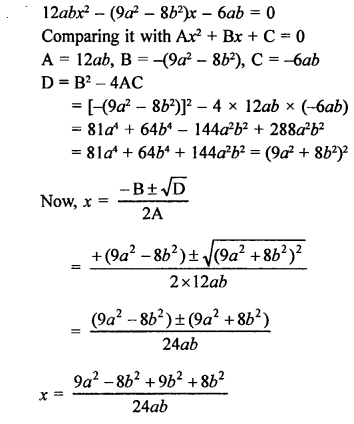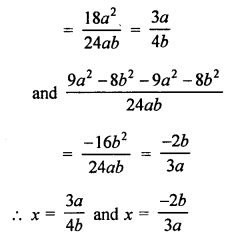Hope given RS Aggarwal Solutions Class 10 Chapter 10 Quadratic Equations Ex 10C are helpful to complete your math homework.

If you have any doubts, please comment below. Learn Insta try to provide online math tutoring for you.